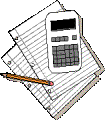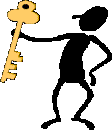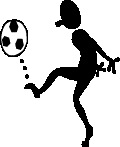A Few words about Solving Problems in Chemistry

Dr. E. T. Bell-LoncellaYou're probably sitting there, wondering, "A hand-out on solving problems? This is a chemistry class, What does she think?" Do you remember word problems from high school math classes? That's the kind of problem! There are several reasons for this handout:

• Most of the problems that you will encounter in this class are what you could call word problems.
• It's very helpful to learn how to solve problems. Solving problems is part of the real world.
• In my years of teaching I've observed students using strategies for solving problems that seem inefficient and ineffective.
• There are many more reasons …

You are also probably wondering what I mean by strategies that are inefficient and ineffective. Three classic behaviors I see are:

• Student reads the problem, and almost immediately starts punching buttons on the calculator.
• Student reads the problem, scribbles a few equations on the page and then looks to see if the answer is in the back of the book.
• Student reads the problem, scribbles a few equations on the page, pushes some buttons on the calculator. Then, exasperated, leans over to the neighbor and says, "What did you get?"

If the problem doesn't have a numerical answer the exasperation escalates. If there isn't an answer in the back of the book ('cause the problem didn't come from the book), the student becomes even more exasperated. Then the student gets upset with the prof, and the class and sometimes will just drops out. It doesn't have to be this way.

Problem solving is a skill and there is a method to solving problems. You will see me model this method in class. You will notice (as students always do) that I rarely will completely solve the problem; I just set it up. This seems to frustrate students. However, I know that you are experts at entering the numbers in your calculators and pushing the Go button. There are several approaches; all of them based on the same idea.

• The most general strategy has only three basic steps:
• First read the problem and look for the information which you have been given. Identify each piece of numerical data in the problem and write it down. Sometimes drawing a sketch is helpful.
• Re-read the problem looking for what the problem wants you to solve. Usually you find this in the sentence that ends with a question mark, or the last sentence. Other keys are words like "determine" and "calculate".
•Now you have to figure out what you need in order to solve the problem. If you haven't already done so, this is a good time to draw a sketch (nothing fancy, just a sketch) of the situation. An outline (or map) of your solution strategy helps. Often, you don't know what the map will look like when you start, but it will become clearer as you work your way though it. You will also want to make a list of necessary conversions, or relevant equations (that become obviously necessary as you develop your solution strategy). When you finally have a pretty clear idea of what your strategy will be, then (and only then) start setting up the equations, plugging in the numbers and (finally) punching them into your calculators.

This approach is very generic and can be applied to any problem … in any discipline. It might seem just a bit too sketchy. The following strategy has a little more structure. It ispresented in a Prep-Chem text, Basic Chemistry: A Problem-Solving Approach(1). It applies the same principles, but adds some extra steps. Gendell begins his discussion of problem solving with a caveat:

A word of advice: begin solving a problem by reviewing the exact meaning of all the terms used, considering the specific physical situation to which the problem refers, and identifying precisely what is asked for in the problem. Do not begin solving a problem by substituting values in one equation or another in the hopes of obtaining the correct answer. It probably is going to take a good deal of work on your part to get used to solving problems in this manner. If you want to be successful in chemistry, however, that is what you will have to do.
As you read the problem the first time, try to create a picture in your mind of the physical situation to which the problem refers. Translate your mental picture into a sketch which you can annotate with physical data (length, width, temp, pressure, etc.)

• Examine your picture and think about they physical principles or mathematical equations that relate the pieces of data provided.
• Make a "map". That is, plan out the necessary calculation steps that will allow you to convert the data provided into the result desired. Gendell suggests that you ask yourself the following series of questions as you devise you "map".
• What quantities are known and what quantities are unknown?
• Are there enough relationships connecting the known and unknown quantities?
• Can the problem be broken down into a series of simpler steps?
• If I want to determine the value of a certain quantity, what other quantities do I need to determine to accomplish this? How do I determine these quantities?
• Use your calculator to carry out the numerical calculations.
• Double check: Is the answer a reasonable one? This is where the skill of estimating is very valuable!
• What did you learn (about solving problems)? How can you apply this to the next (related) problem that you have to solve?

Another strategy is one outlined by Patricia Heller and Mark Hollabaugh who are physics professors.(2) They use five basic steps. See if you can find some similarities between this strategy and the one by Gendell outlined above.

1. Focus the problem - a qualitative description in pictures and (your own) words.
2.Describe the physics (chemistry) - a more complete diagram with the physical (chemical) objects and physical (chemical) quantities as well as the mathematical equations that relate those quantities.
3. Plan the solution - map out the strategy for solving the problem using the equations and other relationships from the previous step (b).
4. Execute the problem - now it's (finally) button-pushing time.
5. Evaluate the answer - is it reasonable and complete?Each of the strategies described thus far is somewhat general - they are basic steps. If you don't feel like you are good at working with something so general, you might want some more specific guidelines. I strongly urge you to read chapter 10 "Solving Chemical Problems: Generic Problems" in the book by Keen and Middlecamp.(3) While it may seem that the ideas they suggest are laborious and take time (especially when you have other subjects to study as well), I believe that if you apply these strategies (to all of your classes, not just to your chemistry) you will find that you will become a better problem-solver overall. (You'll start to have lots of good ideas, just like the little guy to the left there.)

Finally, there are those problems that don't have any numbers and don't necessarily have a right answer. They can be the most daunting and the most challenging. I maintain that it is through striving to solve problems of this sort that you will really learn chemistry. In the introduction to his book Voyages in Conceptual Chemistry the author, Dan Barouch says:As students, please do not just memorize and recite, blindly learning formulas and performing numerous calculations. You must explore and think in order to blossom intellectually. By exploring you will discover, and through discoveries you will learn. Accordingly, this is not merely a problem book. This is not a compendium of recitation exercises, not a long list of numbers to plug into a long list of formulas, not a source of tedious homework sets. In none of these problems will you make numerical calculations. so PUT AWAY YOUR CALCULATORS!! Use these problems as a springboard for conceptual thinking and qualitative understanding. … These problems … ask you to solve puzzles, interpret observations, design experiments and appreciate subtleties in the material. They also challenge you to utilize the versatility of general chemistry in applying your knowledge to solve problems in related subjects that you might study in the future …(4)

Over the course of the semester we will work a wide variety of problems: Drills, problems that bring together ideas form various parts of the Chapter (See Putting it all Together in the end-of-chapter problems in your text book), problems that use lots of mathematical formulas (and equations) and require extensive "button pushing" on your calculator, and conceptual problems taken from Dan Barouch's book. Be sure to keep this handout in a safe place so you can refer to it as needed.

A word about calculators: They can be a curse more than a convenience! Your calculator will give you millions of sig figs; but if you didn't enter the correct number, the sig figs are meaningless. Thus you must learn how to estimate your answers so you can double check that your calculator gave you the correct number! You will not always be allowed to use your calculators during quizzes. The emphasis will be on how well you set up the problem and whether you were able to estimate the answer to the correct number of sig figs. You would b

e well-advised to practice this when working homework problems.

1. Gendell, Julien. Basic Chemistry: A Problem Solving Approach; West: Minneapolis, Mn, 1993.

2. Heller, Patricia; Hollabaugh, Mark "Teaching Problem Solving through Cooperative Grouping. Part 2: Designing Problems and Structuring Gorups. Ame. J. Phys. 1992, 60, 637-645.

3. Kean, Elizabeth; Middlecamp, Catherine How to Survive and Even Excel in General Chemistry; McGraw-Hill: New York, 1994.

4. Barouch, D. H. Voyages in Conceptual Chemistry; Jones and Bartlett: Sudbury , MA, 1997.